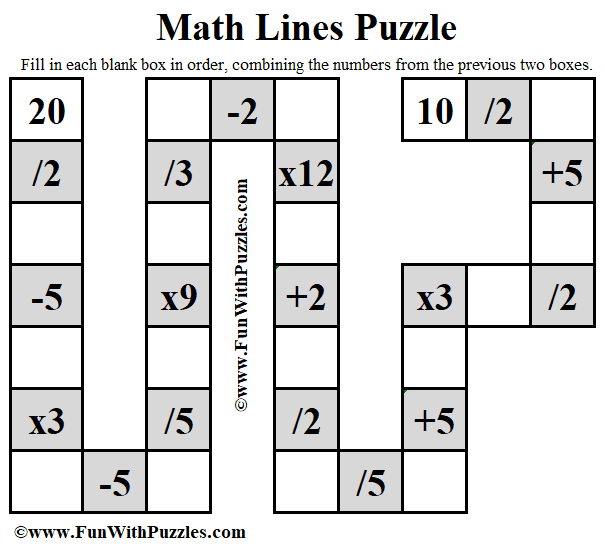This Maths Lines Arithmetic Puzzle is for school-going students. This will test your Mathematical skills. In this puzzle, your task is to calculate a series of Arithmetic Operations to reach the end of the Math Line.Maths Lines Arithmetic Puzzle

You can solve this Math Lines Puzzle in two ways. You can start from the top left number 20 and then reach the other ending number 10 which is on the top right of the puzzle picture
The other way is to start from the top right number 10 and then calculated in reverse to reach the top left number 20. Either way, you will have a different solving experience.